# redgreencmap

Create red and green colormap

## Syntax

``redgreencmap(length)``
``redgreencmap(length,'Interpolation',interpMethod)``

## Description

example

````redgreencmap(length)` returns a `length`-by-`3` matrix containing a red and green colormap. Low values are bright green, values in the center of the map are black, and high values are red. ```

example

````redgreencmap(length,'Interpolation',interpMethod)` specifies the algorithm to use for color interpolation.```

## Examples

collapse all

Read in a sample GPR file.

`madata = gprread('mouse_a1wt.gpr');`

Plot the median foreground intensity for the 635 nm channel.

`maimage(madata,'F635 Median')`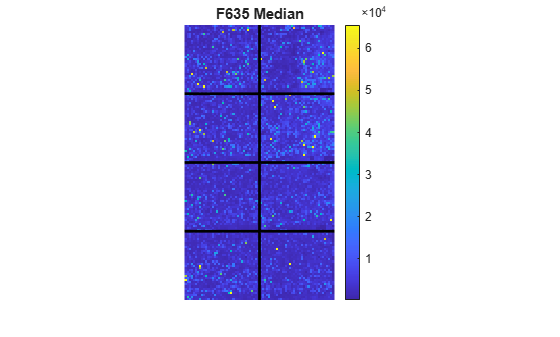Alternatively, create a similar plot using more basic graphics commands.

```F635Median = magetfield(madata,'F635 Median'); figure imagesc(F635Median(madata.Indices));```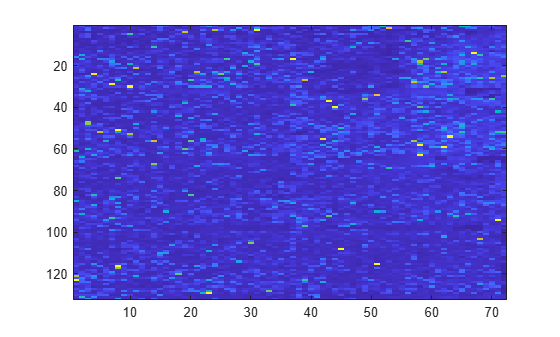Change the colormap to one of the preset colors (for details, see map) and add a color bar.

```colormap('bone') colorbar ```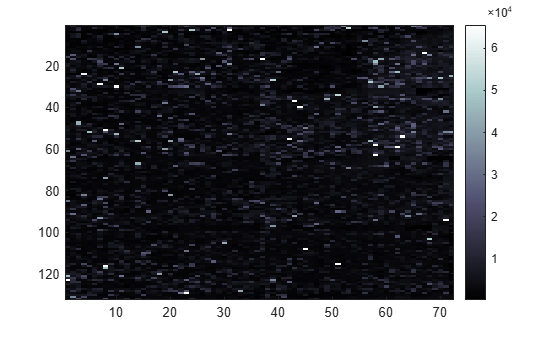Change the colormap to red and green.

`colormap(redgreencmap)`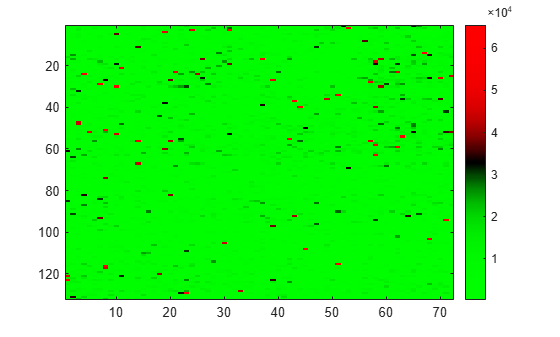You can also change the color interpolation method.

`colormap(redgreencmap(256,'Interpolation','cubic'))`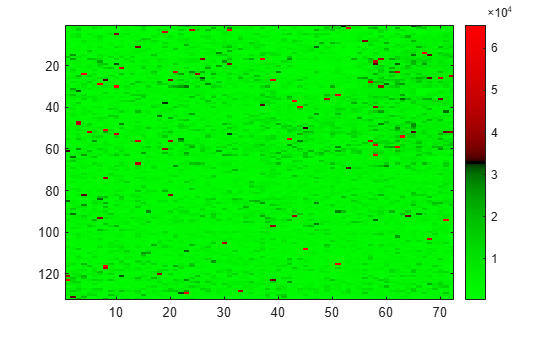Reset the colormap to the default value.

`colormap('default')`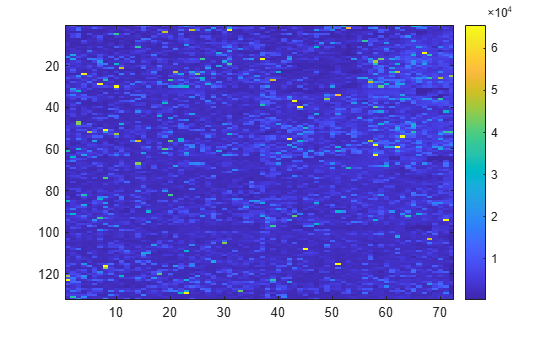## Input Arguments

collapse all

Number of colors in the colormap, specified as empty `[]` or a nonnegative integer. If `length` is empty, the function automatically sets the value to the length of the colormap of the current figure.

Example: `64`

Data Types: `double`

Interpolation algorithm for color interpolation, specified as `'sigmoid'` (which uses `tanh`), `'linear'`, `'quadratic'`, or `'cubic'`.

Example: `'cubic'`

Data Types: `char` | `string`